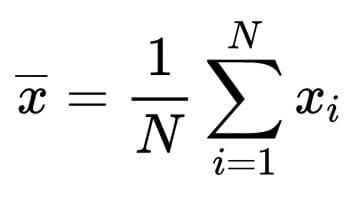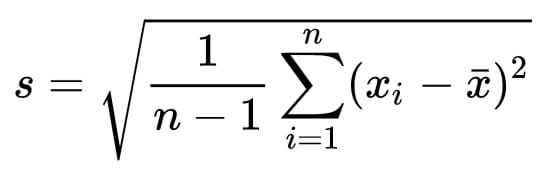﻿

# 統計：一個簡單的樣本變異係數的運算範例

## 題目：請試算（1、2、5、6）四個數值的變異係數。``````1 + 2 + 5 + 6 = 14
14 / 4 = 3.5 = X̄
````````````√(1/4-1)((1-3.5)^2 + (2-3.5)^2 + (5-3.5)^2 + (6-3.5)^2)
√(1/4-1)(6.25 + 2.25 + 2.25 + 6.25)
√(1/3)(17)
√5.666 = 2.38 = s
``````

``````CV = s / X̄ = 2.38 / 3.5 = 0.68
``````